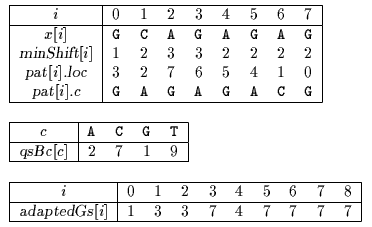Next: Skip Search algorithm Up: ESMAJ Previous: Optimal Mismatch algorithm

# Maximal Shift algorithm

Main features
• variant of the Quick Search algorithm;
• quadratic worst case time complexity;
• preprocessing phase in O(m2+) time and O(m+) space complexity;
• searching phase in O(mn) time complexity.
Description

Sunday designed an algorithm where the pattern characters are scanned from the one which will lead to a larger shift to the one which will lead to a shorter shift. Doing so one may hope to maximize the lengths of the shifts.

The preprocessing phase of the Maximal Shift algorithm consists in sorting the pattern characters in decreasing order of their shift and then in building the Quick Search bad-character shift function (see chapter Quick Search algorithm) and a good-suffix shift function adapted to the scanning order of the pattern characters. It can be done in O(m2+) time and O(m+) space complexity.

The searching phase of the Maximal Shift algorithm has a quadratic worst case time complexity.

The C code

The function preQsBc is given chapter Quick Search algorithm. The functions orderPattern, matchShift and preAdaptedGs are given chapter Optimal Mismatch algorithm.

```typedef struct patternScanOrder {
int loc;
char c;
} pattern;

int minShift[XSIZE];

/* Computation of the MinShift table values. */
void computeMinShift(char *x, int m) {
int i, j;

for (i = 0; i < m; ++i) {
for (j = i - 1; j >= 0; --j)
if (x[i] == x[j])
break;
minShift[i] = i - j;
}
}

/* Maximal Shift pattern comparison function. */
int maxShiftPcmp(pattern *pat1, pattern *pat2) {
int dsh;

dsh = minShift[pat2->loc] - minShift[pat1->loc];
return(dsh ? dsh : (pat2->loc - pat1->loc));
}

/* Maximal Shift string matching algorithm. */
void MS(char *x, int m, char *y, int n) {
pattern pat[XSIZE];

/* Preprocessing */
computeMinShift(x ,m);
orderPattern(x, m, maxShiftPcmp, pat);
preQsBc(x, m, qsBc);

/* Searching */
j = 0;
while (j <= n - m) {
i = 0;
while (i < m && pat[i].c == y[j + pat[i].loc])
++i;
if (i >= m)
OUTPUT(j);
j += MAX(adaptedGs[i], qsBc[y[j + m]]);
}
}

```
The example

Preprocessing phaseTables used by Maximal Shift algorithm.

Searching phase

References
• SUNDAY D.M., 1990, A very fast substring search algorithm, Communications of the ACM . 33(8):132-142.Next: Skip Search algorithm Up: ESMAJ Previous: Optimal Mismatch algorithm

e-mails: {Christian.Charras, Thierry.Lecroq }@laposte.net
Tue Jan 14 15:03:31 MET 1997